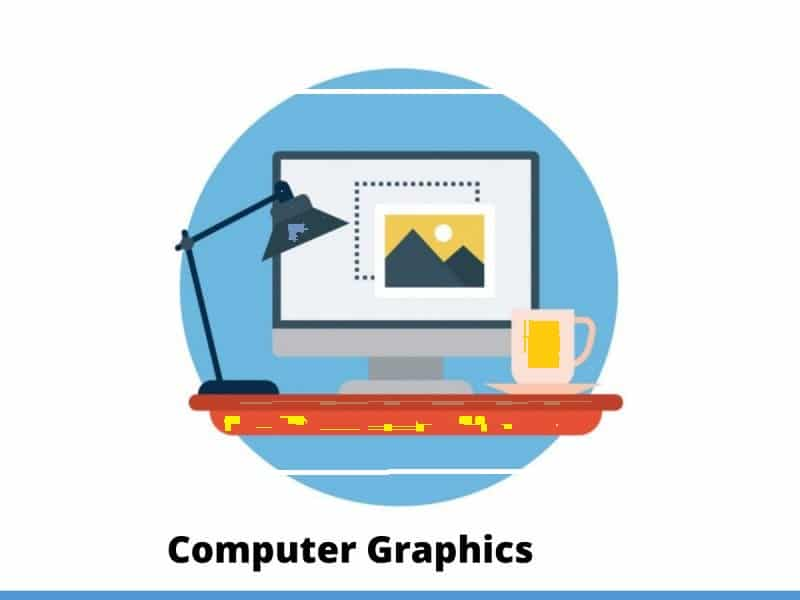## Pointing and Positioning Techniques

Pointing technique refers to look at the items already on the screen whereas the positioning technique refers to position the item on the screen to a new position, i.e., the old current position. The user indicates a position on the screen with an input device, and this position is used to insert a symbol.

There are various pointing and positioning devices which are discussed below:

1. Light Pen
2. Mouse
3. Tablet
4. Joystick
5. Trackball and spaceball

1. Light Pen:

It is a pointing device. When light pen is pointed at an item on the screen, it generates information from which the item can be identified by the program. It does not have any associated tracking hardware instead tracking is performed by software, making use of the output function of the display. All light pen programs depend on a rapid response from the pen when it is pointed at the screen fast response light pens can be build by using a highly sensitive photocell such as a photomultiplier tube.

2. Mouse:

It is a positioning device which consists of a small plastic box resting on two metal wheels whose axes are at right angles. Each wheel of the mouse is linked to a shaft encoder that delivers an electrical pulse for every incremental rotation of the wheel. As the mouse is rolled around on a flat surface, its movement in two orthogonal directions is translated into rotation of the wheels. These rotations can be measured by counting the pulses received from the shaft encoders. The connected values may be held in registers accessible to the computer on written directly into the computer memory. It is simple and low cost, and there is no need to pick it up to use it. The mouse sits on the table surface. But the mouse cannot be used for tracing data from paper since a small rotation of the mouse will cause an error in all the reading and it is complicated handprint character for recognition by the computer.

3. Tablet: It is also a positioning device and is used to describe a flat surface separate from the display, on which the user draws with a stylus. There are two types of tablets:

1. Acoustic Tablet:

It depends on the use of strip microphones which are mounted along two adjacent edges of the tablet. The styles have a small piece of ceramic mounted close to its tip, and at regular intervals, a small spark is generated across the surface of the ceramic between two electrodes. The microphones pick up the pulse of sound produced by the spark and two counters record the delay between creating the spark and receiving the sound. These two delays are proportional to the stylus distance from the two edges of the tablet where the microphones are mounted.

2. Electro-acoustic Tablet:

In this technique, the writing surface is a sheet of magneto strictive material acting as a row of delay lines. An electric pulse travels through the sheet first horizontally and then vertically and is detected by a sensor in the stylus. A counter is used to determine the delay from the time the pulse is issued to the time it is detected; from this value, the position of the stylus can be determined. The electro-acoustic tablet is quieter in operation than its acoustic counterpart and is less affected by noise or air movement.

4. Joystick:

A joystick consists of a small that is used to steer the screen cursor around. The distance that the stick is moved in any direction from its center position corresponds to the screen-cursor movement in that direction. Pressure sensitive joysticks have a non-moveable stick. Pressure on the stick is measured with strain gauges and converted to the movement of the cursor in the direction specified.

5. Trackball and spaceball:

Trackball is a ball that can be rotated with the fingers to produces screen-cursor movement potentiometers, attached to the ball, measure the amount and direction of rotation. Trackballs are after mounted on keyboards, whereas space-ball provides six degrees of freedom. Spaceballs is used for three-dimensional positioning and selection operation in virtual reality system, modeling, animation, CAD and other applications.

### Elastic or Rubber Band Techniques

• Rubber banding is a popular technique of drawing geometric primitives such as line, polylines, rectangle, circle and ellipse on the computer screen.
• It becomes an integral part and de facto standard with the graphical user interface (GUI) for drawing and is almost universally accepted by all windows based applications.
• The user specifies the line in the usual way by positioning its two endpoints. As we move from the first endpoint to the second, the program displays a line from the first endpoint to the cursor position, thus he can see the lie of the line before he finishes positioning it.
• The effect is of an elastic line stretched between the first endpoint and the cursor; hence the name for these techniques.

Consider the different linear structures in fig (a) and fig (d), depending on the position of the cross-hair cursor. The user may move the cursor to generate more possibilities and select the one which suits him for a specific application.### Selection of Terminal Point of the Line:

• The user moves the cursor to the appropriate position and selects.
• Then, as the cursor is moved, the line changes taking the latest positions of the cursors as the end-point.
• As long as the button is held down, the state of the rubber band is active.

The process is explained with the state transition diagram of rubber banding in fig:When the user is happy with the final position, the pressed button is released, and the line is drawn between the start and the last position of the cursor.

Example: This is widely followed in MS-Window based Applications like in the case of a paintbrush drawing package.

Other geometric entities can be drawn in a rubber-band fashion:

• Horizontally or vertically constructed lines
• Rectangles
• Arcs of circles

This technique is very helpful in drawing relatively complex entities such as rectangles and arcs.1. It is used for drawing all geometric entities such as line, polygon, circle, rectangle, ellipse, and other curves.
2. It is easy to understand and implement.

1. It requires computational resources like software and CPU speed.
2. Expensive

### Dragging

Dragging is used to move an object from one position to another position on the computer screen. To drag any other object, first, we have to select the object that we want to move on the screen by holding the mouse button down. As cursor moved on the screen, the object is also moved with the cursor position. When the cursor reached the desired position, the button is released.

The following diagram represents the dragging procedure:### Animation

Animation refers to the movement on the screen of the display device created by displaying a sequence of still images. Animation is the technique of designing, drawing, making layouts and preparation of photographic series which are integrated into the multimedia and gaming products. Animation connects the exploitation and management of still images to generate the illusion of movement. A person who creates animations is called animator. He/she use various computer technologies to capture the pictures and then to animate these in the desired sequence. Animation includes all the visual changes on the screen of display devices. These are:

1. Change of shape as smile, sad and angry etc emoticon.

2. Change in size as very small circle, larger circle and biggest circle etc.
3. Change in color select red unselect white.

4. Change in structure as rotate a triangle.

5. Change in angle as counter clockwise and antilock wise:

### Application Areas of Animation

1. Education and Training:

Animation is used in school, colleges and training centers for education purpose. Flight simulators for aircraft are also animation based.

2. Entertainment:

Animation methods are now commonly used in making motion pictures, music videos and television shows, etc.

One of the best applications of computer animation is Computer Aided Design and is generally referred to as CAD. One of the earlier applications of CAD was automobile designing. But now almost all types of designing are done by using CAD application, and without animation, all these work can’t be possible.

This is one of the significant applications of computer animation. The most important advantage of an animated advertisement is that it takes very less space and capture people attention.

5. Presentation:

Animated Presentation is the most effective way to represent an idea. It is used to describe financial, statistical, mathematical, scientific & economic data.

### Animation Functions

1. Morphing:

Morphing is an animation function which is used to transform object shape from one form to another is called Morphing. It is one of the most complicated transformations. This function is commonly used in movies, cartoons, advertisement, and computer games. Example: Face App make one younger image into older.

The process of Morphing involves three steps:

1. In the first step, one initial image and other final image are added to morphing application as shown in fig: Ist & 4th object consider as key frames.
2. The second step involves the selection of key points on both the images for a smooth transition between two images as shown in 2nd object.3. In the third step, the key point of the first image transforms to a corresponding key point of the second image as shown in 3rd object of the figure.

2. Wrapping:

Wrapping function is similar to morphing function. It distorts only the initial images so that it matches with final images and no fade occurs in this function.
3. Tweening:

Tweening is the short form of ‘inbetweening.’ Tweening is the process of generating intermediate frames between the initial & last final images. This function is popular in the film industry.4. Panning:

Usually Panning refers to rotation of the camera in horizontal Plane. In computer graphics, Panning relates to the movement of fixed size window across the window object in a scene. In which direction the fixed sized window moves, the object appears to move in the opposite direction as human walk but the moon in fix plane but seem to us moving with us. Another example running car and rain.

If the window moves in a backward direction, then the object appear to move in the forward direction and the window moves in forward direction then the object appear to move in a backward direction.

5. Zooming:

In zooming, the window is fixed an object and change its size, the object also appear to change in size. When the window is made smaller about a fixed center, the object comes inside the window appear more enlarged. This feature is known as Zooming In.

When we increase the size of the window about the fixed center, the object comes inside the window appear small. This feature is known as Zooming Out.

6. Fractals:

Fractal Function is used to generate a complex picture by using Iteration. Iteration means the repetition of a single formula again & again with slightly different value based on the previous iteration result. These results are displayed on the screen in the form of the display picture.## Introduction of Transformations

Computer Graphics provide the facility of viewing object from different angles. The architect can study building from different angles i.e.

1. Front Evaluation
2. Side elevation
3. Top plan

A Cartographer can change the size of charts and topographical maps. So if graphics images are coded as numbers, the numbers can be stored in memory. These numbers are modified by mathematical operations called as Transformation.

The purpose of using computers for drawing is to provide facility to user to view the object from different angles, enlarging or reducing the scale or shape of object called as Transformation.

1. Each transformation is a single entity. It can be denoted by a unique name or symbol.
2. It is possible to combine two transformations, after connecting a single transformation is obtained, e.g., A is a transformation for translation. The B transformation performs scaling. The combination of two is C=AB. So C is obtained by concatenation property.

There are two complementary points of view for describing object transformation.

1. Geometric Transformation: The object itself is transformed relative to the coordinate system or background. The mathematical statement of this viewpoint is defined by geometric transformations applied to each point of the object.
2. Coordinate Transformation: The object is held stationary while the coordinate system is transformed relative to the object. This effect is attained through the application of coordinate transformations.

An example that helps to distinguish these two viewpoints:

The movement of an automobile against a scenic background we can simulate this by

• Moving the automobile while keeping the background fixed-(Geometric Transformation)
• We can keep the car fixed while moving the background scenery- (Coordinate Transformation)

1. Translation
2. Scaling
3. Rotating
4. Reflection
5. Shearing

### Translation

It is the straight line movement of an object from one position to another is called Translation. Here the object is positioned from one coordinate location to another.

### Translation of point:

To translate a point from coordinate position (x, y) to another (x1 y1), we add algebraically the translation distances Tx and Ty to original coordinate.

x1=x+Tx
y1=y+Ty

The translation pair (Tx,Ty) is called as shift vector.

Translation is a movement of objects without deformation. Every position or point is translated by the same amount. When the straight line is translated, then it will be drawn using endpoints.

For translating polygon, each vertex of the polygon is converted to a new position. Similarly, curved objects are translated. To change the position of the circle or ellipse its center coordinates are transformed, then the object is drawn using new coordinates.

Let P is a point with coordinates (x, y). It will be translated as (x1 y1).#### Matrix for Translation:### Scaling:

It is used to alter or change the size of objects. The change is done using scaling factors. There are two scaling factors, i.e. Sx in x direction Sy in y-direction. If the original position is x and y. Scaling factors are Sx and Sy then the value of coordinates after scaling will be x1 and y1.

If the picture to be enlarged to twice its original size then Sx = Sy =2. If Sxand Sy are not equal then scaling will occur but it will elongate or distort the picture.

If scaling factors are less than one, then the size of the object will be reduced. If scaling factors are higher than one, then the size of the object will be enlarged.

If Sxand Syare equal it is also called as Uniform Scaling. If not equal then called as Differential Scaling. If scaling factors with values less than one will move the object closer to coordinate origin, while a value higher than one will move coordinate position farther from origin.

Enlargement: If T1=,If (x1 y1)is original position and T1is translation vector then (x2 y2) are coordinated after scalingThe image will be enlarged two timesReduction: If T1=. If (x1 y1) is original position and T1 is translation vector, then (x2 y2) are coordinates after scaling#### Matrix for Scaling:Example: Prove that 2D Scaling transformations are commutative i.e, S1 S2=S2 S1.

Solution: S1 and S2 are scaling matrices### Rotation:

It is a process of changing the angle of the object. Rotation can be clockwise or anticlockwise. For rotation, we have to specify the angle of rotation and rotation point. Rotation point is also called a pivot point. It is print about which object is rotated.

#### Types of Rotation:

1. Anticlockwise
2. Counterclockwise

The positive value of the pivot point (rotation angle) rotates an object in a counter-clockwise (anti-clockwise) direction. The negative value of the pivot point (rotation angle) rotates an object in a clockwise direction. When the object is rotated, then every point of the object is rotated by the same angle.

Straight Line: Straight Line is rotated by the endpoints with the same angle and redrawing the line between new endpoints.

Polygon: Polygon is rotated by shifting every vertex using the same rotational angle.

Curved Lines: Curved Lines are rotated by repositioning of all points and drawing of the curve at new positions.

Circle: It can be obtained by center position by the specified angle.

Ellipse: Its rotation can be obtained by rotating major and minor axis of an ellipse by the desired angle.Matrix for rotation is a clockwise direction.Matrix for rotation is an anticlockwise direction.Matrix for homogeneous co-ordinate rotation (clockwise)Matrix for homogeneous co-ordinate rotation (anticlockwise)#### Rotation about an arbitrary point:

If we want to rotate an object or point about an arbitrary point, first of all, we translate the point about which we want to rotate to the origin. Then rotate point or object about the origin, and at the end, we again translate it to the original place. We get rotation about an arbitrary point.

Example: The point (x, y) is to be rotated

The (xc yc) is a point about which counterclockwise rotation is done

Step1: Translate point (xc yc) to originStep2: Rotation of (x, y) about the originStep3: Translation of center of rotation back to its original positionExample1: Prove that 2D rotations about the origin are commutative i.e. R1 R2=R2 R1.

Solution: R1 and R2are rotation matrices### Reflection:

It is a transformation which produces a mirror image of an object. The mirror image can be either about x-axis or y-axis. The object is rotated by180°.

#### Types of Reflection:

3. Reflection about an axis perpendicular to xy plane and passing through the origin

1. Reflection about x-axis: The object can be reflected about x-axis with the help of the following matrixIn this transformation value of x will remain same whereas the value of y will become negative. Following figures shows the reflection of the object axis. The object will lie another side of the x-axis.2. Reflection about y-axis: The object can be reflected about y-axis with the help of following transformation matrixHere the values of x will be reversed, whereas the value of y will remain the same. The object will lie another side of the y-axis.

The following figure shows the reflection about the y-axis3. Reflection about an axis perpendicular to xy plane and passing through origin:
In the matrix of this transformation is given belowIn this value of x and y both will be reversed. This is also called as half revolution about the origin.

4. Reflection about line y=x: The object may be reflected about line y = x with the help of following transformation matrixFirst of all, the object is rotated at 45°. The direction of rotation is clockwise. After it reflection is done concerning x-axis. The last step is the rotation of y=x back to its original position that is counterclockwise at 45°.

Example: Find reflected position of triangle i.e., to the x-axis.

Solution:The a point coordinates after reflectionThe b point coordinates after reflectionThe coordinate of point c after reflectiona (3, 4) becomes a1 (3, -4)
b (6, 4) becomes b1 (6, -4)
c (4, 8) becomes c1 (4, -8)

### Shearing:

It is transformation which changes the shape of object. The sliding of layers of object occur. The shear can be in one direction or in two directions.

#### Shearing in the X-direction:

In this horizontal shearing sliding of layers occur. The homogeneous matrix for shearing in the x-direction is shown below:Shearing in the Y-direction: Here shearing is done by sliding along vertical or y-axis.Shearing in X-Y directions:

Here layers will be sided in both x as well as y direction. The sliding will be in horizontal as well as vertical direction. The shape of the object will be distorted. The matrix of shear in both directions is given by:### Composite Transformation:

A number of transformations or sequence of transformations can be combined into single one called as composition. The resulting matrix is called as composite matrix. The process of combining is called as concatenation. Suppose we want to perform rotation about an arbitrary point, then we can perform it by the sequence of three transformations

1. Translation
2. Rotation
3. Reverse Translation

The ordering sequence of these numbers of transformations must not be changed. If a matrix is represented in column form, then the composite transformation is performed by multiplying matrix in order from right to left side. The output obtained from the previous matrix is multiplied with the new coming matrix.

#### Example showing composite transformations:

The enlargement is with respect to center. For this following sequence of transformations will be performed and all will be combined to a single one. Composition of two Scaling: The composition of two scaling is multiplicative. Let S11 and S12are matrix to be multiplied.Step1: The object is kept at its position as in fig (a)

Step2: The object is translated so that its center coincides with the origin as in fig (b)

Step3: Scaling of an object by keeping the object at origin is done in fig (c)

Step4: Again translation is done. This second translation is called a reverse translation. It will position the object at the origin location.

Above transformation can be represented as TV.STV-1#### Note: Two types of rotations are used for representing matrices one is column method. Another is the row method.#### Advantage of composition or concatenation of matrix:

1. It transformations become compact.
2. The number of operations will be reduced.
3. Rules used for defining transformation in form of equations are complex as compared to matrix.

#### Composition of two translations:

Let t1 t2 t3 t4are translation vectors. They are two translations P1 and P2. The matrix of P1 and P2 given below. The P1 and P2are represented using Homogeneous matrices and P will be the final transformation matrix obtained after multiplication.Above resultant matrix show that two successive translations are additive.

#### Composition of two Rotations: Two Rotations are also additive

Composition of two Scaling: The composition of two scaling is multiplicative. Let S11 and S12are matrix to be multiplied.## Scan Conversion on Line Generation Algorithm

Scan Conversion defer  is a process of representing graphics objects a collection of pixels that is 1(on set) or 0 (offset). In general, a line consist of connecting two points. It is a basic  in computer graphics. In order to draw a line, you need two points between which you can draw a line.

### Scan Converting a Straight Line

A straight line may be defined by two endpoints & an equation. In fig the two endpoints are described by (x1,y1) and (x2,y2). The equation of the line is used to determine the x, y coordinates of all the points that lie between these two endpoints.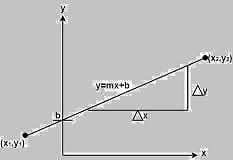Using the equation of a straight line, y = mx + b where m =& b = the y interrupt, we can find values of y by incrementing x from x =x1, to x = x2. By scan-converting these calculated x, y values, we represent the line as a sequence of pixels.

### Properties of Good Line Drawing Algorithm:

1. Line should appear Straight: We must appropriate the line by choosing addressable points close to it. If we choose well, the line will appear straight, if not, we shall produce crossed lines.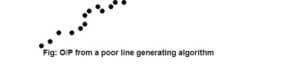The lines must be generated parallel or at 45° to the x and y-axes. Other lines cause a problem: a line segment through it starts and finishes at addressable points, may happen to pass through no another addressable points in between.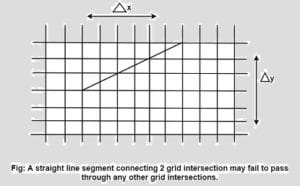2. Lines should terminate accurately: Unless lines are plotted accurately, they may terminate at the wrong place.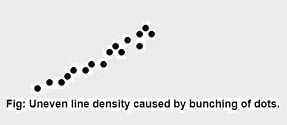3. Lines should have constant density: Line density is proportional to the no. of dots displayed divided by the length of the line. In order to maintain constant density, dots should be equally spaced.

4. Line density should be independent of line length and angle: This can be done by computing an approximating line-length estimate and to use a line-generation algorithm that keeps line density constant to within the accuracy of this estimate.

5. Line should be drawn rapidly: This computation should be performed by special-purpose hardware.

### Algorithm for line Drawing:

1. Direct use of line equation
2. DDA (Digital Differential Analyzer)
3. Bresenham’s Algorithm

### Direct use of line equation:

It is the simplest form of conversion. First of all scan P1 and P2 points. P1 has co-ordinates (x1‘,y1‘) and (x2‘ y2‘ ).

Then       m = (y2‘,y1‘)/( x2‘,x1‘) and b =If value of |m|≤1 for each integer value of x. But do not considerIf value of |m|>1 for each integer value of y. But do not considerExample: A line with starting point as (0, 0) and ending point (6, 18) is given. Calculate value of intermediate points and slope of line.

Solution: P1 (0,0) P7 (6,18)

x1=0
y1=0
x2=6
y2=18We know equation of line is
y =m x + b
y = 3x + b…………..equation (1)

put value of x from initial point in equation (1), i.e., (0, 0) x =0, y=0
0 = 3 x 0 + b
0 = b ⟹ b=0

put b = 0 in equation (1)
y = 3x + 0
y = 3x

Now calculate intermediate points

Let x = 1 ⟹ y = 3 x 1 ⟹ y = 3
Let x = 2 ⟹ y = 3 x 2 ⟹ y = 6
Let x = 3 ⟹ y = 3 x 3 ⟹ y = 9
Let x = 4 ⟹ y = 3 x 4 ⟹ y = 12
Let x = 5 ⟹ y = 3 x 5 ⟹ y = 15
Let x = 6 ⟹ y = 3 x 6 ⟹ y = 18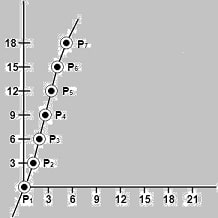So points are

P1 (0,0);  P2 (1,3) ; P3 (2,6); P4 (3,9); P5 (4,12); P6 (5,15); P7 (6,18)

### Algorithm for drawing line using equation:

Step1: Start Algorithm

Step2: Declare variables x1,x2,y1,y2,dx,dy,m,b,

Step3: Enter values of x1,x2,y1,y2.
The (x1,y1) are co-ordinates of a starting point of the line.
The (x2,y2) are co-ordinates of a ending point of the line.

Step4: Calculate dx = x2– x1

Step5: Calculate dy = y2-y1

Step6: Calculate m =Step7: Calculate b = y1-m* x1

Step8: Set (x, y) equal to starting point, i.e., lowest point and xendequal to largest value of x.

If dx < 0
then x = x2
y = y2
xend= x1
If dx > 0
then x = x1
y = y1
xend= x2

Step9: Check whether the complete line has been drawn if x=xend, stop

Step10: Plot a point at current (x, y) coordinates

Step11: Increment value of x, i.e., x = x+1

Step12: Compute next value of y from equation y = mx + b

Step13: Go to Step9.

### Program to draw a line using Line Slope Method

#include <graphics.h>
#include <stdlib.h>
#include <math.h>
#include <stdio.h>
#include <conio.h>
#include <iostream>

using namespace std;

class bresen
{
float x, y, x1, y1, x2, y2, dx, dy, m, c, xend;
public:
void get ();
void cal ();
};
int main ()
{
bresen b;
b.get ();
b.cal ();
getch ();
}
void bresen :: get ()
{
printf (“Enter start & end points”);
printf (“enter x1, y1, x2, y2\n”);
scanf (“%f%f%f%f”,&x1, &y1, &x2, &y2);
}
void bresen ::cal ()
{
/* request auto detection */
int gdriver = DETECT,gmode, errorcode;
/* initialize graphics and local variables */
initgraph (&gdriver, &gmode, ” “);
/* read result of initialization */
errorcode = graphresult();
if (errorcode != grOk) /* an error occurred */ /*an error occurred */
{
printf(“Graphics error: %s\n”, grapherrormsg(errorcode));
printf(“Press any key to halt:”);
getch ();
exit (1); /* terminate with an error code */
}
dx = x2-x1;
dy=y2-2*y1;
m = dy/dx;
c = y1 – (m * x1);
if (dx<0)
{
x=x2;
y=y2;
xend=x1;
}
else
{
x=x1;
y=y1;
xend=x2;
}
while (x<=xend)
{
putpixel (x, y, RED);
y++;
y=(x*x) +c;
}
}

OUTPUT:

Enter Starting and End Points
Enter (X1, Y1, X2, Y2)
200 100 300 200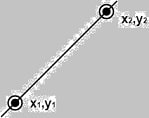In general, a line consist of connecting two points. It is a basic  in computer graphics. In order to draw a line, you need two points between which you can draw a line.

In the following three algorithms, we refer the one point of line as X0,Y0X0,Y0 and the second point of line as X1,Y1X1,Y1.

### DDA Algorithm(Digital Differential Analyzer )

DDA algorithm is the simple line generation algorithm which is detailed step by step here.

Step 1 − Get the input of two end points (X0,Y0)(X0,Y0) and (X1,Y1)(X1,Y1).

Step 2 − Calculate the difference between two end points.

dx = X1 - X0
dy = Y1 - Y0

Step 3 − Based on the calculated difference in step-2, you need to identify the number of steps to put pixel. If dx > dy, then you need more steps in x coordinate; otherwise in y coordinate.

if (absolute(dx) > absolute(dy))
Steps = absolute(dx);
else
Steps = absolute(dy);

Step 4 − Calculate the increment in x coordinate and y coordinate.

Xincrement = dx / (float) steps;
Yincrement = dy / (float) steps;


Step 5 − Put the pixel by successfully incrementing x and y coordinates accordingly and complete the drawing of the line.

for(int v=0; v < Steps; v++)
{
x = x + Xincrement;
y = y + Yincrement;
putpixel(Round(x), Round(y));
}

### Bresenham’s Line Generation

Bresenham’s Line Algorithm  is used for scan converting a line. It was developed by Bresenham. It is an incremental scan conversion algorithm. The big advantage of this algorithm is that, it uses only integer calculations. Moving across the x axis in unit intervals and at each step choose between two different y coordinates. It is an efficient method because it involves only integer addition, subtractions, and multiplication operations. These operations can be performed very rapidly so lines can be generated quickly. In this method, next pixel selected is that one who has the least distance from true line.

### The method works as follows:

Assume a pixel P1′(x1′,y1′),then select subsequent pixels as we work our may to the night, one pixel position at a time in the horizontal direction toward P2′(x2′,y2′). Once a pixel in choose at any step. The next pixel is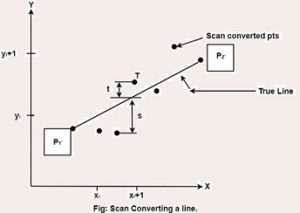Either the one to its right (lower-bound for the line). One top its right and up (upper-bound for the line) The line is best approximated by those pixels that fall the least distance from the path between P1′,P2′.

### Bresenham’s Line Algorithm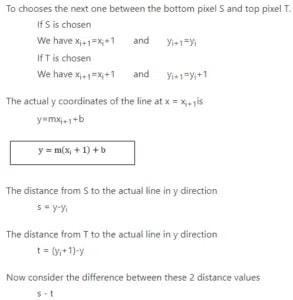When (s-t) <0 ⟹ s < t

The closest pixel is S

When (s-t) ≥0 ⟹ s < t

The closest pixel is T

This difference is
s-t = (y-yi)-[(yi+1)-y]
= 2y – 2yi -1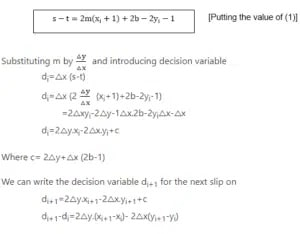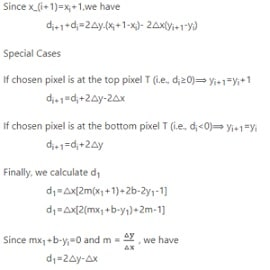1. It involves only integer arithmetic, so it is simple.

2. It avoids the generation of duplicate points.

3. It can be implemented using hardware because it does not use multiplication and division.

4. It is faster as compared to DDA (Digital Differential Analyzer) because it does not involve floating point calculations like DDA Algorithm.

1. This algorithm is meant for basic line drawing only Initializing is not a part of Bresenham’s line algorithm. So to draw smooth lines, you should want to look into a different algorithm.

### Bresenham’s Line Algorithm:

Step1: Start Algorithm

Step2: Declare variable x1,x2,y1,y2,d,i1,i2,dx,dy

Step3: Enter value of x1,y1,x2,y2
Where x1,y1are coordinates of starting point
And x2,y2 are coordinates of Ending point

Step4: Calculate dx = x2-x1
Calculate dy = y2-y1
Calculate i1=2*dy
Calculate i2=2*(dy-dx)
Calculate d=i1-dx

Step5: Consider (x, y) as starting point and xendas maximum possible value of x.
If dx < 0
Then x = x2
y = y2
xend=x1
If dx > 0
Then x = x1
y = y1
xend=x2

Step6: Generate point at (x,y)coordinates.

Step7: Check if whole line is generated.
If x > = xend
Stop.

Step8: Calculate co-ordinates of the next pixel
If d < 0
Then d = d + i1
If d ≥ 0
Then d = d + i2
Increment y = y + 1

Step9: Increment x = x + 1

Step10: Draw a point of latest (x, y) coordinates

Step11: Go to step 7

Step12: End of Algorithm

### Example: Starting and Ending position of the line are (1, 1) and (8, 5). Find intermediate points.

Solution: x1=1
y1=1
x2=8
y2=5
dx= x2-x1=8-1=7
dy=y2-y1=5-1=4
I1=2* ∆y=2*4=8
I2=2*(∆y-∆x)=2*(4-7)=-6
d = I1-∆x=8-7=1

 x y d=d+I1 or I2 1 1 d+I2=1+(-6)=-5 2 2 d+I1=-5+8=3 3 2 d+I2=3+(-6)=-3 4 3 d+I1=-3+8=5 5 3 d+I2=5+(-6)=-1 6 4 d+I1=-1+8=7 7 4 d+I2=7+(-6)=1 8 5

### Bresenham’s Line Algorithm

Program to implement Bresenham’s Line Drawing Algorithm:

#include<stdio.h>
#include<graphics.h>
void drawline(int x0, int y0, int x1, int y1)
{
int dx, dy, p, x, y;
dx=x1-x0;
dy=y1-y0;
x=x0;
y=y0;
p=2*dy-dx;
while(x<x1)
{
if(p>=0)
{
putpixel(x,y,7);
y=y+1;
p=p+2*dy-2*dx;
}
else
{
putpixel(x,y,7);
p=p+2*dy;}
x=x+1;
}
}
int main()
{
int gdriver=DETECT, gmode, error, x0, y0, x1, y1;
initgraph(&gdriver, &gmode, "c:\\turboc3\\bgi");
printf("Enter co-ordinates of first point: ");
scanf("%d%d", &x0, &y0);
printf("Enter co-ordinates of second point: ");
scanf("%d%d", &x1, &y1);
drawline(x0, y0, x1, y1);
return 0;
}

Output: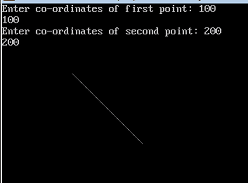Bresenham’s Line Algorithm

### Differentiate between DDA Algorithm and Bresenham’s Line Algorithm:

DDA Algorithm Bresenham’s Line Algorithm
1. DDA Algorithm use floating point, i.e., Real Arithmetic. 1. Bresenham’s Line Algorithm use fixed point, i.e., Integer Arithmetic
2. DDA Algorithms uses multiplication & division its operation 2.Bresenham’s Line Algorithm uses only subtraction and addition its operation
3. DDA Algorithm is slowly than Bresenham’s Line Algorithm in line drawing because it uses real arithmetic (Floating Point operation) 3. Bresenham’s Algorithm is faster than DDA Algorithm in line because it involves only addition & subtraction in its calculation and uses only integer arithmetic.
4. DDA Algorithm is not accurate and efficient as Bresenham’s Line Algorithm. 4. Bresenham’s Line Algorithm is more accurate and efficient at DDA Algorithm.
5.DDA Algorithm can draw circle and curves but are not accurate as Bresenham’s Line Algorithm, it can draw circle and curves with more accurate.

### Mid-Point Algorithm

Mid-point algorithm is due to Bresenham which was modified by Pitteway and Van Aken. Assume that you have already put the point P at x,yx,y coordinate and the slope of the line is 0 ≤ k ≤ 1 as shown in the following illustration. Now you need to decide whether to put the next point at E or N. This can be chosen by identifying the intersection point Q closest to the point N or E. If the intersection point Q is closest to the point N then N is considered as the next point; otherwise E.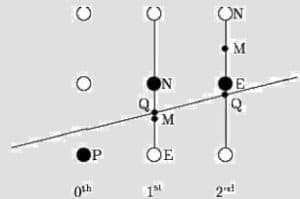To determine that, first calculate the mid-point Mx+1,y+½x+1,y+½. If the intersection point Q of the line with the vertical line connecting E and N is below M, then take E as the next point; otherwise take N as the next point. In order to check this, we need to consider the implicit equation:

Fx,yx,y = mx + b – y

For positive m at any given X,

• If y is on the line, then Fx,yx,y = 0
• If y is above the line, then Fx,yx,y < 0
• If y is below the line, then Fx,yx,y > 0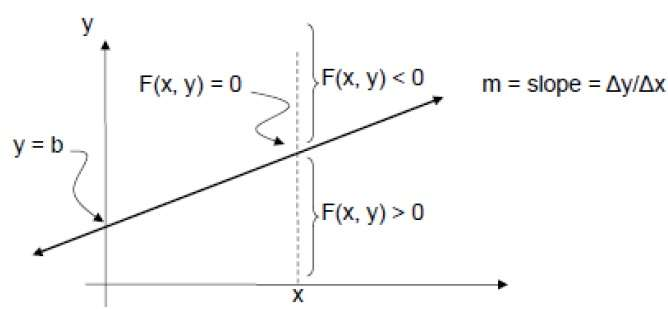## Introduction of Computer Graphics

Generally, it is difficult to display an image of any size on the computer screen. This method is simplified by using Computer graphics. Graphics on the computer are produced by using various algorithms and techniques. This tutorial describes how a rich visual experience is provided to the user by explaining how all these processed by the computer. It involves technology to access. The Process transforms and presents information in a visual form. The role of computer graphics insensible. In today life, computer graphics has now become a common element in user interfaces, T.V. commercial motion pictures. Computer Graphics is the creation of pictures with the help of a computer. The end product of the computer graphics is a picture it may be a business graph, drawing, and engineering. In computer graphics, two or three-dimensional pictures can be created that are used for research. Many hardware devices algorithm has been developing for improving the speed of picture generation with the passes of time. It includes the creation storage of models and image of objects. These models for various fields like engineering, mathematical and so on.

### Definition of Computer Graphics:

It is the use of computers to create and manipulate pictures on a display device. It comprises of software techniques to create, store, modify, represents pictures.

### Why computer graphics used?

Suppose a shoe manufacturing company want to show the sale of shoes for five years. For this vast amount of information is to store. So a lot of time and memory will be needed. This method will be tough to understand by a common man. In this situation graphics is a better alternative. Graphics tools are charts and graphs. Using graphs, data can be represented in pictorial form. A picture can be understood easily just with a single look. Interactive computer graphics work using the concept of two-way communication between computer users. The computer will receive signals from the input device, and the picture is modified accordingly. Picture will be changed quickly when we apply command.

### Types of Computer Graphics

Raster Graphics: In raster graphics pixels are used for an image to be drawn. It is also known as a bitmap image in which a sequence of image is into smaller pixels. Basically a bitmap indicates a large number of pixels together.
Vector Graphics: In vector graphics, mathematical formulae are used to draw different types of shapes, lines, objects and so on.

### Computer Graphics Applications:

• Computer Art: MS Paint.
• Presentation Graphics : It is used to summarize financial statistical scientific or economic data. For example- Bar chart, Line chart.
• Entertainment: It is used in motion picture, music video, television gaming.
• Education and training: It is used to understand operations of complex system. It is also used for specialized system such for framing for captains, pilots and so on.
• Visualization:  To study trends and patterns. For example: Analyzing satellite photo of earth.
• Computer graphics user interfaces GUIs :A graphic, mouse-oriented paradigm which allows the user to interact with a computer. For engineering and architectural system, these are used in electrical automobile, electro-mechanical, mechanical, electronic devices. For example: gears and bolts.
• Business presentation graphics :A picture is worth a thousand words”.
• Cartography: Drawing maps.
• Weather Maps :Real-time mapping, symbolic representations.
• Satellite Imaging: Geodesic images.
• Photo Enhancement: Sharpening blurred photos.
• Medical imaging :MRIs, CAT scans, etc. – Non-invasive internal examination.
• Engineering drawings: mechanical, electrical, civil, etc. – Replacing the blueprints of the past.
• Typography: The use of character images in publishing – replacing the hard type of the past.
• Architecture :Construction plans, exterior sketches – replacing the blueprints and hand drawings of the past.
• Art: Computers provide a new medium for artists.
• Training: Flight simulators, computer aided instruction, etc.
• Simulation and modeling: Replacing physical modeling and enactments

### Cathode Ray Tube

The video monitor is  primary output device in a graphical system. The main element of a video monitor is the Cathode Ray Tube CRTCRT, shown in the following illustration.

The operation of CRT is very simple:

• The electron gun emits a beam of electrons cathode rays.
• The electron beam passes through focusing and deflection systems that direct it towards specified positions on the phosphor coated screen.
• When the beam hits the screen, the phosphor emits a small spot of light at each position contacted by the electron beam.
• It redraws the picture by directing the electron beam back over the same screen points quickly.

There are two ways Random scan and Raster scan by which we can display an object on the screen.

### Raster Scan

In a raster scan system, the electron beam is swept across the screen, one row at a time from top to bottom. As the electron beam moves across each row, the beam intensity is turned on and off to create a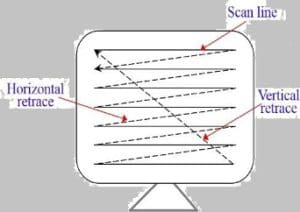pattern of illuminated spots.

Picture definition is stored in memory area called the Refresh Buffer or Frame Buffer. This memory area holds the set of intensity values for all the screen points. Stored intensity values are then retrieved from the refresh buffer and “painted” on the screen one row scanline at a time as shown in the following illustration.  Each screen point is referred to as a pixel picture element or pel. At the end of each scan line, the electron beam returns to the left side of the screen to begin displaying the next scan line.

### Random Scan Vector Scan

In this technique, the electron beam is directed only to the part of the screen where the picture is to be drawn rather than scanning from left to right and top to bottom as in raster scan. It is also called vector display, stroke-writing display, or calligraphic display. Picture definition is stored as a set of line-drawing commands in an area of memory referred to as the refresh display file. To display a specified picture, the system cycles through the set of commands in the display file, drawing each component line in turn. After all the line-drawing commands are processed, the system cycles back to the first line command in the list. Random-scan displays are designed to draw all the component lines of a picture 30 to 60 times each second.

### Scan Conversion

Scan Conversion  is a process of representing graphics objects a collection of pixels. The graphics objects are continuous. The pixels used are discrete. Each pixel can have either on or off state. The circuitry of the video display device of the computer is capable of converting binary values (0, 1) into a pixel on and pixel off information. 0 is represented by pixel off. 1 is represented using pixel on. Using this ability graphics computer represent picture having discrete dots. Any model of graphics can be reproduced with a dense matrix of dots or points. Most human beings think graphics objects as points, lines, circles, ellipses. For generating graphical object, many algorithms have been developed. Examples of objects which can be scan

• converted
• Point
• Line
• Sector
• Arc
• Ellipse
• Rectangle
• Polygon
• Characters
• Filled Regions

The process of converting is also called as rasterization. The algorithms implementation varies from one computer system to another computer system. Some algorithms are implemented using the software, hardware or firmware.

## Pixel or Pel:

pixel is a short form of the picture element. that is also called a point or dot. It is the smallest picture unit accepted by display devices. A picture is constructed from hundreds of such pixels. Pixels are generated using commands. Lines, circle, arcs, characters; curves are drawn with closely spaced pixels. In order to display the digit or letter matrix of pixels is used.The coordinate is represented using row and column. P (5, 5) used to represent a pixel in the 5th row and the 5th column. Each pixel has some intensity value which is represented in memory of computer called a frame buffer. Frame Buffer is also called a refresh buffer.

### Scan Converting a Point

Each pixel on the graphics display does not represent a mathematical point. Rather, it means a region which theoretically can contain an infinite number of points. Scan-Converting a point involves illuminating the pixel that contains the point.

Example: Display coordinates pointsas shown in fig would both be represented by pixel (2, 1). In general, a point p (x, y) is represented by the integer part of x & the integer part of y that is pixels [(INT (x), INT (y).